• 实验报告 课程名称 《算法分析与设计》 实验日期 2020 年 3 月 10日 至 2020年 3 月 17 日 学生姓名 戴昊宇 所在班级 计算机184 学号 2018212212015 实验名称 检索算法(二分查找法) 实验地点 ...
实验报告
课程名称	  《算法分析与设计》                  	实验日期	2020  年 3  月 10日 至  2020年 3 月  17 日
学生姓名	   戴昊宇                 	所在班级	   计算机184             	学号	   2018212212015
实验名称	   检索算法(二分查找法)
实验地点	                              	同组人员	      无
1.问题
在一个排好序的数组T[1…n]中查找x，如果x在T中，输出x在T的下标j；如果x不在T中，输出j=0.按实验模板编写，“分析”部分仅给出复杂度结果即可。
2.解析
用二分查找法进行数组检索。
3.设计
输入：数组T，下标从 l 到 r；数 x
输出：j     // 如果 x 在 T 中，j为下标；否则为0
1.  l<-1; r<-n
2.  while l<= r do
3.       m<-[(l+r)/2]
4.       if T[m]=x  then return m 　 // x恰好等于中位元素
5.       else if T[m]>m  then r<-m-1
6.              else <-m+1
7.      return 0
4.分析
时间复杂度:O(logn)
5.源码
https://github.com/yyqx-1128/text


展开全文• 实验内容：二分查找 三．实验目的：掌握查找法的工作原理及应用过程，利用其工作原理完成上述实验题目中的内容。 四．实验要求：为了使学生更好的掌握与理解课堂上老师所讲的概念与原理，实验前每个学生要认真预习...
• 简单的初级菜鸟上传的数据结构试验报告，我是大二学的数据结构，主要是帮助那些一点都不会的更菜的菜鸟~！
• 实验报告 课程名称：《算法分析与设计》 实验日期： 2021年 3 月 8 日 至 2021年3月15日 学生姓名：cyx 所在班级：计算机194 学号 12305 实验名称： Prim算法构造最小生成树 Kruskal算法构造最小生成树 实验...
1. 问题
写出两种检索算法：在一个排好序的数组T[1…n]中查找x，如果x在T中，输出x在T的下标j；如果x不在T中，输出j=0.按实验模板编写，“分析”部分仅给出复杂度
结果即可
2. 解析3. 设计

void SequenceSearch(int key, int n) {//顺序查找
for (int i = 1; i <= n; i++)
if (t[i] == key)
{
cout << "利用顺序查找成功，下标为" << i << endl;
return;
}
cout << "查找失败";
return;
}
void BinarySearch(int key, int n) {//二分查找
int left = 1;
int right = n;
while (left <= right) {
int m = (left + right) / 2;
if (key == t[m]) {
cout << "二分查找成功下标为：" << m << endl;
return;
}
else if (key < t[m])
right = m - 1;
else
left = m + 1;
}
cout << "查找失败";
return;
}


4. 分析
顺序查找：时间复杂度为O(n)
二分查找：时间复杂度为O(log2n)
5. 源码
https://github.com/moshang1113/SearchAlgorithm/blob/main/%E6%BA%901.cpp


展开全文• python实现递归算法 ...实验内容 问题1，实现 fibonacci 的递归和非递归。要求计算F（100）的值，比较两种方法的性能要求1）有合适的提示从键盘输入数据；例如“Please input the number to calculate:...
python实现递归算法

一、开发环境

开发工具：jupyter notebook 并使用vscode，cmd命令行工具协助编程测试算法
编程语言：python3.6

二、实验内容

问题1，实现 fibonacci 的递归和非递归。要求计算F（100）的值，比较两种方法的性能要求1）有合适的提示从键盘输入数据；例如“Please input the number to calculate:”2）有输出结果（下同）

Fibonacci非常有名，这里就不多介绍了，直接实现代码。

非递归版本实现的fibonacci数列代码：

# 实现fibonacci的递归算法以及非递归算法
# fibonacco数列前几项 为了检验结果是否正确 1 1 2 3 5 8 13 21 34

# 使用非递归建立fibonacci数列
def fibonacci(n):
a = 1
b = 1
if n==1 or n==2:p
return 1
else:
for i in range(n-2):
a, b = b, a+b
return b

if __name__ == '__main__':
import time
n = int(input("Please input the number to calculate:"))
start = time.time()
print(fibonacci(n))
end = time.time()
print("非递归版本的运行时间为", end-start)


利用jupyter notebook的运行工具进行运行，运行的出的结果为：递归版本实现的fibonacci数列代码（效率低下版本）

# 低效的做法
def fibonacci_recursion(n):
if n<=2:
return 1
else:
return fibonacci_recursion(n-1)+fibonacci_recursion(n-2)


这个版本的代码实现起来非常容易，并且十分易懂，不过缺点就是效率实在是太低了，当输入100的时候，计算了相当长的时间，所以考虑换一种思路，按照非递归版本的方法，从前依推导出后面的数据。

递归版本实现的fibonacci数列代码（换思路的版本）

# 使用递归建立fibonacci数列
def fibonacci_recursion(n, a=1, b=1, c=3):
if n < 3:
return 1
else:
if n==c:
return a+b
else:
return fibonacci_recursion(n, a=b, b=a+b, c=c+1)

if __name__ == '__main__':
import time
n = int(input("Please input the number to calculate:"))
start = time.time()
print(fibonacci_recursion(n))
end = time.time()
print("递归版本的运行时间为", end-start)


这一版本的代码虽然比起上面的复杂了点，但是效率还是非常快的。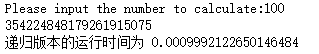EXTRA

上面的版本可以说是挺好的了，然而当我输入2000的时候，还是报错了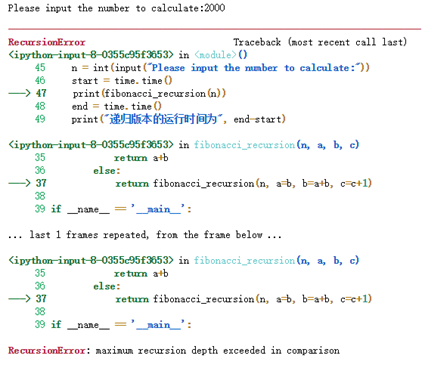好像说是超过了最大递归的深度，爆栈了。

依稀地记得，以前写代码的时候有过这种问题的解决方案，于是开始百度。

然而尴尬的是找了半天，并没有找到我上次的那份代码，上次的好像是用上了装饰器解决问的，不过查到了另外的解决方案，使用尾递归优化，并使用了python的生成器机制。代码如下：

# python 使用尾递归优化
import types    # 导入types模块
# 如果函数中出现了yield解释器会自动将函数当成生成器，此时是不会直接生成数的
# tramp的作用为：使生成器不断产生下一个数据
def tramp(gen, *args, **kwargs):
g = gen(*args, **kwargs)
while isinstance(g, types.GeneratorType):   # 如果g为生成器的实例
g=g.__next__()  #
return g

def fibonacci_recursion(n, a=1, b=1, c=3):
if n < 3:
yield 1
else:
if n==c:
yield a+b
else:
yield fibonacci_recursion(n, a=b, b=a+b, c=c+1)

if __name__ == '__main__':
import time
n = int(input("Please input the number to calculate:"))
start = time.time()
print(fibonacci_recursion(n))
end = time.time()
print("递归版本的运行时间为", end-start)


网上找到的代码是py2的版本的，最近都是用的py3，版本问题还真是头疼呀，花了点时间改了一下。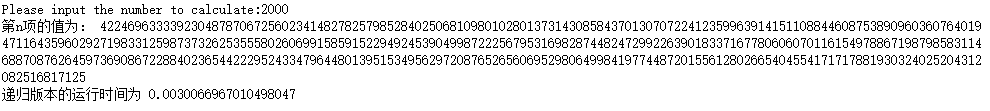经过优化的递归函数效果惊人,2000以内的都不在是问题啦。

问题2,实现全排列算法

实现前20个奇数的全排列。
实现数字1，3，5，7，9五个数字的全排列
实现对数字1,3,5,7,9五个数字的全排列

# 实现全排列的算法。
# a) 实现数字1，3，5，7，9五个数字的全排列。
# b) 实现前20个奇数的全排列。

# 全排列函数
def full_arrangement(lis):
length = len(lis)
if length < 2:    # 边界条件
return lis
else:
result = []
for i in range(length):
ch = lis[i]    # 取出str中每一个字符
rest = lis[0: i] + lis[i+1: length]
for s in full_arrangement(rest):  # 递归
result.append(ch+s) # 将ch与子问题的解依次组合
return result

if __name__ == '__main__':
lis = [1, 3, 5, 7, 9]
lis1 = list(map(str, lis))

import time
start = time.time()
print(full_arrangement(lis1))
end = time.time()
print("全排列运行时间：", end-start)


对五个数进行全排列的运行时间：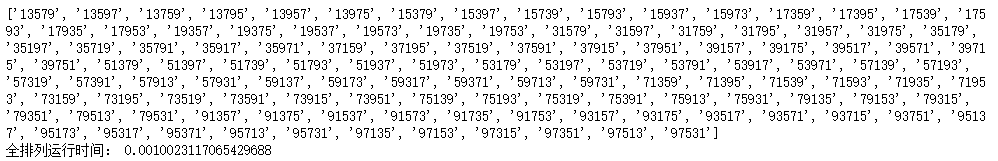下一个问题：实现前20个奇数的全排列。

测试代码已经写好了，是这个样子的

lis2 = list(map(str, [x for x in range(40) if x%2!=0]))
print(lis2)
start = time.time()
print(full_arrangement(lis2))
end = time.time()
print("全排列运行时间：", end-start)


然而，emmm……，好像运行时间太长了点。

当对前10个奇数进行全排列的时候，运行结果如下图所示：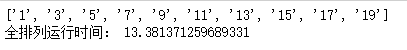10个数花了13秒的时间，可见前20个奇数所用时间非常的长，然而现在并没有找到更好地优化解决方案，只能实现到这个地步，感到非常抱歉。

问题3，实现二分查找。

在 1 3 3 4 5 5 7 8 8 9 10 中查找得到7，返回其所在的位置。
随机生成10000个整数，查找数字 2025，返回其所在的位置。
原理：

折半查找法也称为二分查找法，它充分利用了元素间的次序关系，采用分治策略，可在最坏的情况下用O(log n)完成搜索任务。它的基本思想是，将n个元素分成个数大致相同的两半，取a[n/2]与欲查找的x作比较，如果x=a[n/2]则找到x，算法终止。如 果x<a[n/2]，则我们只要在数组a的左半部继续搜索x（这里假设数组元素呈升序排列）。如果x>a[n/2]，则我们只要在数组a的右半部继续搜索x。

要求：

1.必须采用顺序存储结构。

2.必须按关键字大小有序排列。

代码实现：

# 实现二分查找。
# a) 在 1 3 3 4 5 5 7 8 8 9 10 中查找得到7，返回其所在的位置。
# b) 随机生成10000个整数，查找数字 2025，返回其所在的位置。
import random
# 二分查找，如果在lis中找到n，则返回n的下标，如果找不到，则返回-1
def binary_search(lis, n):
length = len(lis)

left = 0
right = length-1
while left <= right:
mid = (left+right)//2
# 注意，注意，注意
# 这里要注意，py3使用//作为整除，好像程老师的书《算法分析与设计》P69页存在这个错误
if lis[mid] == n:
return mid
elif lis[mid] > n:
right = mid-1
elif lis[mid] < n:
left = mid+1
return -1

if __name__ == '__main__':
lis =  [1, 3, 3, 4, 5, 5, 7, 8, 8, 9, 10]
print('数字7的下标为:',binary_search(lis, 7))

# 随机生成10000个成递增的数据
x = 0
lis2 = []
for i in range(10000):
lis2.append(x)
x += int(random.random()*10)

find = binary_search(lis2, 2026)
if find == -1:
print('没有找到2026！')
else:
print('找到2026，位置为', find)


多次运行情况：

运行one运行two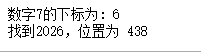问题4，实现合并排序和快速排序，比较算法的性能。

输入数据为：3 2 5 7 8 9 5 0 1

随机生成10000个整数。

实现归并排序还有快速排序

归并排序的实现方式还是记得的，写起来也毫无压力，关于快速排序这个，以前用python写过两遍，可惜的是……全部在边界数据上失败了……快速排序果然还是有点难度的，就记得个大概的方法，所以这次的快速排序是照着我一起用C++写的版本写出来的，重新用python实现了一遍。

# 实现合并排序和快速排序，比较算法的性能。
# a) 输入数据为：3 2 5 7 8 9 5 0 1
# b) 随机生成10000个整数。
import numpy as np
import random
import time

# 将两个有序列表合并，归并排序的辅助函数
def merge(lis1, lis2):
i = 0
j = 0
lis3 = [] # 合并之后的列表
while i < len(lis1) and j < len(lis2):
if lis1[i] < lis2[j]:
lis3.append(lis1[i])
i += 1
else:
lis3.append(lis2[j])
j += 1
# 处理剩下的数据
while i < len(lis1):
lis3.append(lis1[i])
i += 1
while j < len(lis2):
lis3.append(lis2[j])
j += 1
return lis3

# 归并排序 分解列表操作
def merge_sort(lis):
if len(lis) <= 1:
return lis
middle = len(lis)//2

leftlis = lis[:middle]
rightlis = lis[middle: ]
left_sorted = merge_sort(leftlis)
right_sorted = merge_sort(rightlis)
return merge(left_sorted, right_sorted)

# 快速排序，划分操作， 快速排序的辅助函数
def split(lis, first, last):
pivot = lis[first]

left = first
right = last
while left<right:
while pivot < lis[right]:
right=right-1
while left < right and (lis[left] < pivot or lis[left] == pivot):
left=left+1
if left < right:
lis[left], lis[right] = lis[right], lis[left]
# 确定好基准位置
pos = right
lis[first] = lis[pos]
lis[pos] = pivot
return pos

# 快速排序实现
def quicksort(lis, first, last):
if first < last:
pos = split(lis, first, last)
quicksort(lis, first, pos-1)
quicksort(lis, pos+1, last)
return lis

if __name__ == '__main__':
lis1 = [3, 2, 5, 7, 8, 9, 5, 0, 1]
lis2 = [3, 2, 5, 7, 8, 9, 5, 0, 1]
print('使用归并排序:',merge_sort(lis))
print('使用快速排序:',quicksort(lis, 0, len(lis)-1))

# 生成两个一样的10000个随机数的列表, 使用deepcopy函数
lis1 = [ int(random.random()*10000) for i in range(10000)]
import copy
lis2 = copy.deepcopy(lis1)	# 深度拷贝

# 测试运行时间
start = time.time()
#     print(merge_sort(lis1))  # 打印排序的数据
merge_sort(lis1)
end = time.time()
print('使用快速排序，排10000个随机数的时间:', end-start)

start = time.time()
#     print(quicksort(lis2, 0, len(lis2)-1)) # 打印排序的数据
quicksort(lis2, 0, len(lis2)-1)
end = time.time()
print('使用快速排序，排10000个随机数的时间:', end-start)



运行的结果：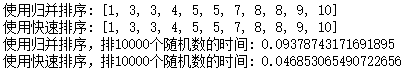这样看来，果然还是快速排序排的比较快呀~~~

三、实验总结

这次的实验内容以前全部用C++写过，现在代码还留着，所以这次使用了python语言全部重写一遍，感觉收获还是很多的，不仅使我重新巩固了有关算法方面的知识，还学会了很多新的知识和python技巧，比如优化递归的尾递归优化，py的cProfile性能分析还有itertools迭代工具模块等等，我再次深刻体会到学习算法是一件事情，把算法实现写出又是另外一件事情，也许某个算法的道理很简单，但是实现和编程过程还是需要很多的技巧的，看着写实验报告这么辛苦，于是决定把它放在博客上，就是这样。
展开全文算法 python
• /* (程序头部注释开始) ...* 文件名称：字典（二分查找） * 作 者： 刘镇 * 完成日期： 2012 年 06 月 05日 * 版 本 号： 1.078 * 对任务及求解方法的描述部分 * 输入描述： ...... *


/* (程序头部注释开始)
* 程序的版权和版本声明部分
* Copyright (c) 2012, 烟台大学计算机学院学生
* All rights reserved.
* 文件名称：字典（二分查找）

* 作 者： 刘镇
* 完成日期： 2012 年 06 月 05日
* 版 本 号： 1.078

* 对任务及求解方法的描述部分
* 输入描述： ......

* 问题描述： ......

* 程序输出： ......

* 程序头部的注释结束
*/
#include<fstream>

#include<iostream>

#include<iomanip>

#include<string>

using namespace std;

class Dictionary
{
public:
void write_dictionary(ifstream & in);
string get_e();
string get_c();
string get_w();
private:
string e;
string c;
string w;
};

void Dictionary::write_dictionary(ifstream & in)
{
in >> this->e >> this->c >> this->w;
}

void writefile(Dictionary * d, int num)
{
ifstream infile( "dictionary.txt", ios::in );

if(!infile)
{
cerr << "open error" << endl;

exit(1);
}
for(int i = 0; i < num; ++i)
{
d[i].write_dictionary(infile);
}

infile.close();
}

string Dictionary::get_e()
{
return this->e;
}

string Dictionary::get_c()
{
return this->c;
}

string Dictionary::get_w()
{
return this->w;
}

void search(Dictionary * d, int num)
{

string find_word;

do
{
int low = 0;
int mid = 0;
int high = num - 1;
bool flag = false;

cout << "请输入您要查找的英文单词: " << endl;

cin >> find_word;

while(low <= high)
{
mid = (low + high) / 2;

if(d[mid].get_e() == find_word)
{
cout << d[mid].get_e() << '\t';
cout << d[mid].get_w() << '\t';
cout << d[mid].get_c() << endl;
flag = true;
break;
}
if(d[mid].get_e() < find_word)
{
low = mid + 1;
}
else
{
high = mid - 1;
}
}

if(flag == false && find_word != "0000")
{
cout << "未找到该单词！" << endl;
}

}while(find_word != "0000");
}

int main()
{
Dictionary dic;
string find_word;

writefile(dic, 8000);
search(dic, 8024);

system("pause");
return 0 ;
}  

运行结果：与上篇博文无太大差别

缺点：不知道为啥要在search（）中数字为8024

心得体会：

二分查找当初在做上学期题目的字典时觉得是挺麻烦的，但是经过下学期的提高，真的感觉到理解能力提高了，在百度上打上二分查找，读一段代码，即可解决问题。用书面语言讲就是折半法，将你所用的分段查找，精确定位，省去了要从头找到位的麻烦。但由于实现排序，才能实现分割，也带来了不便于插入的缺点。

提供我学习的一个代码（重在体会）：http://wenku.baidu.com/view/4632e62acfc789eb172dc836.html

感言：

这个问题就只有几个难点：二分查找算一个（较简单），对类的处理数据成员（三个字符串数组）一个，文件读入一个（将其做成函数）


展开全文任务 c++ dictionary string search
• 算法分析与设计实验报告 ...1.使用二分搜索算法查找有序数列中的指定元素； 2.通过上机实验进行算法实现； 3.保存和打印出程序的运行结果，并结合程序进行分析，上交实验报告。 算法描述及实验步骤 实验步骤：算法 java
• 算法实现1顺序查找读入文件中的数据查找一个key统计时间 // 2二分查找读入文件排序二分查找key统计时间 // 3分块查找读入文件分100块每块300+数字查找key统计时间 // 4二分查找树读入文件形成BST查找key统计时间 //...
• 算法第二章上机实验报告 软工1802 20181003080 吴子熙 实验题目一： 7-1二分查找(20分) 输入n值(1<=n<=1000)、n个非降序排列的整数以及要查找的数x，使用二分查找算法查找x，输出x所在的...
• 实验报告要求任选一题进行分析。内容包括： 实践题目 问题描述 算法描述 算法时间及空间复杂度分析（要有分析过程） 心得体会（对本次实践收获及疑惑进行总结） 本次实践一共三道题，我选择第一道题来分析...
• 安徽大学本科课程《算法设计与分析》实验二《二分查找算法BinarySearch》，包括.m文件和实验报告
• 一、PTA实验报告题1 ：二分查找 　1.1　实践题目 　1.2　问题描述 　1.3　算法描述 　1.4　算法时间及空间复杂度分析 二、PTA实验报告题2 ：改写二分搜索算法 　2.1　实践题目 　2.2　问题描述 　2.3　算法...
• 实验名称: 二分搜索算法 实验地点： 实验目的： 理解分治算法的概念和... 二分搜索算法也称为折半查找法，它充分利用了元素间的次序关系，采用分治策略，可在最坏的情况下用O(log n)完...C++ 算法
• 1、实践题目：二分查找 2、问题描述：输入n值(1<=n<=1000)、n个非降序排列的整数以及要查找的数x，使用二分查找算法查找x，输出x所在的下标（0~n-1）及比较次数。若x不存在，输出-1和比较次数。 3、算法描述...
• 1 实践题目:二分查找 2 问题描述： 输入n值(1<=n<=1000)、n个非降序排列的整数以及要查找的数x，使用二分查找算法查找x，输出x所在的下标（0~n-1）及比较次数。若x不存在，输出-1和比较次数。 3 算法描述 ...
• 报告介绍了了遍历查找和二分查找两种算法，并用 python 语言将其实现。通过对要查找的数组的大小的变化控制，用 matplotlib 库函数绘制出了两种算法分别的时间复杂度的图像，并进行数据分析和比较。 关键词： 遍历...算法
• 1 掌握查找的不同方法，并能用高级... 2 熟练掌握顺序表和有序表的顺序查找和二分查找方法。 3 掌握排序的不同方法，并能用高级语言实现排序算法。 4 熟练掌握顺序表的选择排序、冒泡排序和直接插入排序算法的实现。
• 一共四项实验：大数乘法+最近点对问题+最优二分查找树+所有点的最短路径，详细报告可以参考：http://www.doc88.com/p-1814881556545.html
• 一．实验题目，设计思路 1.实验题目 　题1：11-6方阵循环右移（20） ...　本题要求编写程序，将给定n...　题2：12-5查找指定字符（15） 　本题要求编写程序，从给定字符串中查找某指定的字符。 　题...
• 1.使用二分搜索算法查找任意N个有序数列中的指定元素。 2.通过上机实验进行算法实现。 3.保存和打印出程序的运行结果，并结合程序进行分析，上交实验报告。 4.至少使用两种方法进行编程，直接查找/递归查找/迭代查找...